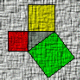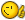# Append 3D Points to a List

## Recommended PostsI have tried several combination and gone thru about a 100 posts but I can't figure out what I am doing wrong.

I am filtering thru objects and pulling out a 3D coordinate from the database. Then I am turning that into a 3 point list (x y z). Then I want to create a list of those points and add to them to the existing list as the program filters thru the remaining objects from the selection set.

```(progn
(setq unitpoint_orig (vl-string-right-trim ";" (substr (vlax-get entvla 'points) 12)))
(if (and(or (= Z_pref "Y")(= Z_pref "y"))(/= Z_height nil))
(progn
(setq unitpoint_mod (comma_pull unitpoint_orig "REAL_NUM"))
)
(setq unitpoint (comma_pull unitpoint_orig "REAL_NUM"))
)
(setq unitpoint_lst (append unitpoint_lst 'unitpoint))

;(command "insert" pl_block unitpoint "1" "1" "0")
)
```

Current Result: (105.13 3165.22 0.0 105.13 3194.65 0.0 105.13 3142.5 0.0)

Desired Result: ((105.13 3165.22 0.0)(105.13 3194.65 0.0)(105.13 3142.5 0.0))

Once I get this part working, I am then planning on filtering for duplicates using Lee Mac's subroutines. Then inserting based off those points.

##### Share on other sitesTheyCallMeJohn.

You could use this small routine on your list.

```(defun tupl3 (l) (if l (cons (list (car l) (cadr l) (caddr l))(tupl3 (cdddr l)))))
```

So for example:

```(tupl3 '(105.13 3165.22 0.0 105.13 3194.65 0.0 105.13 3142.5 0.0))

((105.13 3165.22 0.0) (105.13 3194.65 0.0) (105.13 3142.5 0.0))
_\$
```

or change

```(setq unitpoint_lst (append unitpoint_lst 'unitpoint))

to

(setq unitpoint_lst (append unitpoint_lst (list unitpoint)))
```

##### Share on other sitesExactly what I needed...( I swear I tired the same thing with " ' " instead of "list" but I guess I made a mistake,

TheyCallMeJohn.

```(setq unitpoint_lst (append unitpoint_lst 'unitpoint))

to

(setq unitpoint_lst (append unitpoint_lst (list unitpoint)))
```

##### Share on other sitesNot a big deal with small lists, but ( cons ) is a ton faster than ( append ).

You basically (cons pt list) and then if the order of the list is important (reverse lst)

If you work with say 30,000 atoms, it can make a significant difference. -David

-David

##### Share on other sitesTheyCallMeJohn,

David is exactly right, I tend to stay away from append.

In your case you could simply :

```(setq unitpoint_lst (cons unitpoint unitpoint_lst))

; and if necessary at the end :

(setq unitpoint_lst (reverse unitpoint_lst))
```

Also ,not entirely necessary but, I usually set the list

to nil before starting to build it. It is specially

useful in the debugging phase where very often

you have not declared your variables and are

running test.

ymg

##### Share on other sitesTheyCallMeJohn,

David is exactly right, I tend to stay away from append.

In your case you could simply :

```(setq unitpoint_lst (cons unitpoint unitpoint_lst))

; and if necessary at the end :

(setq unitpoint_lst (reverse unitpoint_lst))
```

Also ,not entirely necessary but, I usually set the list

to nil before starting to build it. It is specially

useful in the debugging phase where very often

you have not declared your variables and are

running test.

ymg

David and Ymg,

Thanks for the suggestion. I will try out cons and see if that works. Also I do set it to nil, I just put it right under the defun so when I am done it easier to make the necessary variables local once I debug the code.

UPDATE:

So it tried substituting this:

`(setq unitpoint_lst (cons unitpoint_lst (list unitpoint)))`

for this:

`(setq unitpoint_lst (append unitpoint_lst (list unitpoint)))`

And it didn't work, is there something else I need to change?

Edited by TheyCallMeJohn
Update

##### Share on other sitesTheyCallMeJohn,

Here is a small example making use of Gilles Chanteau's parsing routine.

I have setup a dummy list of string to parse:

```(defun test ()
(setq datalist '(";;;1.0, 2.0, 3.0,       weerertttretqrt"  ";;;4.0, 5.0, 6.0,       weerertttretqrt"    ";;;7.0, 8.0,9.0,       weerertttretqrt"))
(setq parsedlist nil)
(foreach item datalist
(setq parsedlist (cons (str2lst (vl-string-left-trim ";" item) ",") parsedlist))
)
(setq coordlist nil)
(foreach str parsedlist
(setq coordlist (cons (list (atof (car str)) (atof (cadr str)) (atof (caddr str))) coordlist))
)

(princ)
)

;; str2lst     by Gilles Chanteau                                             ;
;; Transform a string with separator into a list of strings.                  ;
;;                                                                            ;
;; Arguments:                                                                 ;
;; str : string of characters                                                 ;
;; sep : separator characters                                                 ;

(defun str2lst (str sep / len lst)
(setq len (strlen sep))
(while (setq pos (vl-string-search sep str))
(setq lst (cons (substr str 1 pos) lst)
str (substr str (+ len pos 1))
)
)
(reverse (cons (substr str 1 pos) lst))
)
```

Now here are the reult after running:

```_\$ (test)
_\$ parsedlist
(("7.0" " 8.0" "9.0" "       weerertttretqrt") ("4.0" " 5.0" " 6.0" "       weerertttretqrt") ("1.0" " 2.0" " 3.0" "       weerertttretqrt"))
_\$ coordlist
((1.0 2.0 3.0) (4.0 5.0 6.0) (7.0 8.0 9.0))
_\$
```

As you can see as you go through the first foreach loop, your result come out with

the last item at the first position.

Now we run another foreach loop to cons the list of coordinates and you end up

with the list in the correct order.

So say we did not have to do the second loop, but you still want your list in the proper

order. You woul issue (reverse list).

The only reason we are telling you to use cons is that it is faster than append by a factor of ten

specially on long list. In your case you don't have to, but it is nonetheless something you

want to know.

Now if you would post your typical data line as returned by (vlax-get entvla 'points),

maybe we could come up with suggestion to make your task easier.

ymg

##### Share on other sitesTheytCallMeJohnn,

In answer to post #6 what you need is:

`(setq unitpoint_lst (cons unitpoint  unitpoint_list))`

ymg

## Join the conversation

You can post now and register later. If you have an account, sign in now to post with your account.
Note: Your post will require moderator approval before it will be visible.×   Pasted as rich text.   Paste as plain text instead

Only 75 emoji are allowed.